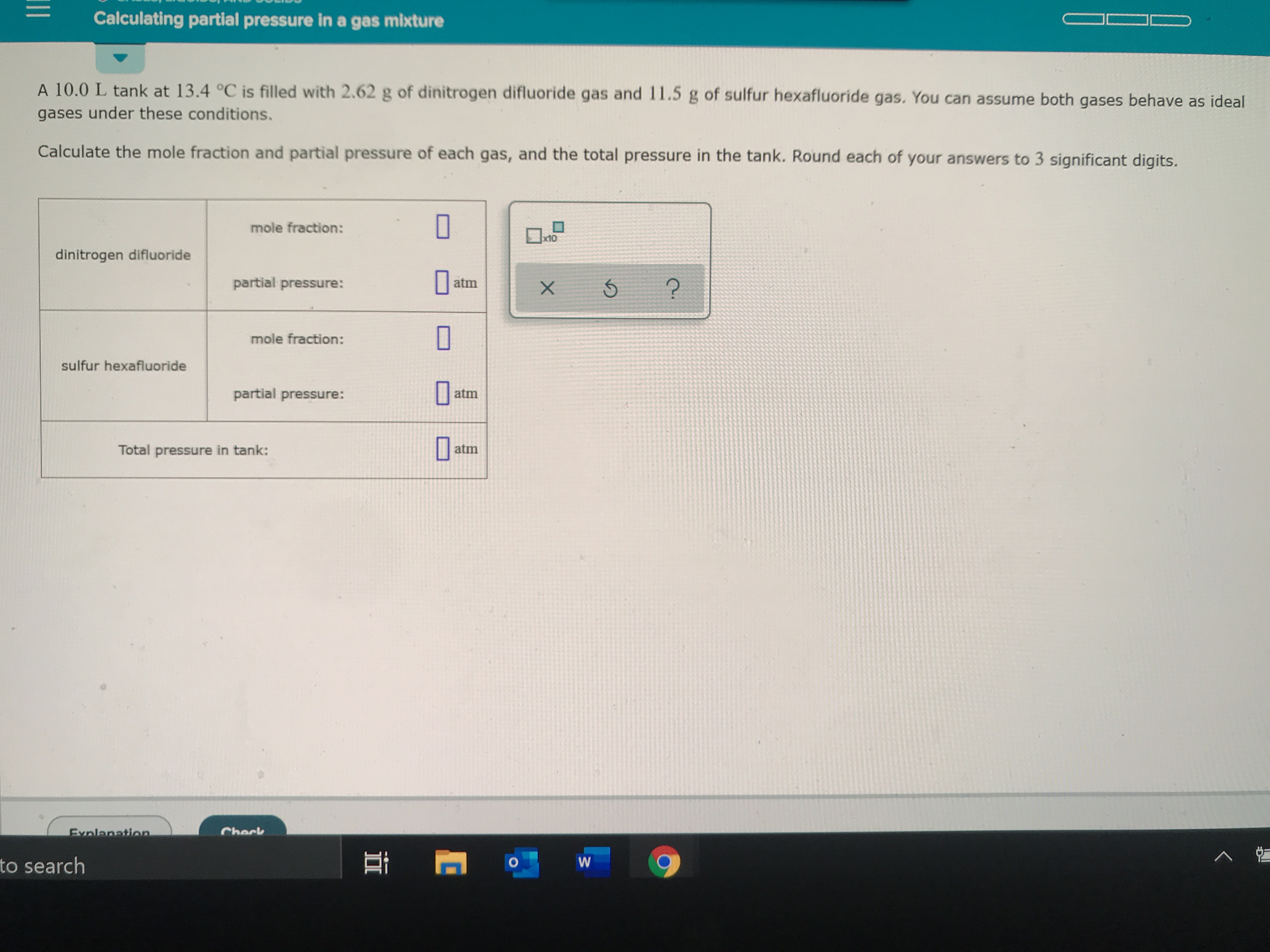# Calculating partial pressure in a gas mixtureA 10.0 L tank at 13.4 °C is filled with 2.62 g of dinitrogen difluoride gas and 11.5 g of sulfur hexafluoride gas. You can assume both gases behave as idealgases under these conditions.Calculate the mole fraction and partial pressure of each gas, and the total pressure in the tank. Round each of your answers to 3 significant digits.mole fraction:x10dinitrogen difluoridepartial pressure:atmmole fraction:sulfur hexafluoridepartial pressure:atmatmTotal pressure in tank:CheckExnlanationto searchWX

Question
67 views

Calculate the mole fraction and partial pressure of each gas and the total pressure in the tank.help_outlineImage TranscriptioncloseCalculating partial pressure in a gas mixture A 10.0 L tank at 13.4 °C is filled with 2.62 g of dinitrogen difluoride gas and 11.5 g of sulfur hexafluoride gas. You can assume both gases behave as ideal gases under these conditions. Calculate the mole fraction and partial pressure of each gas, and the total pressure in the tank. Round each of your answers to 3 significant digits. mole fraction: x10 dinitrogen difluoride partial pressure: atm mole fraction: sulfur hexafluoride partial pressure: atm atm Total pressure in tank: Check Exnlanation to search W X fullscreen
check_circle

star
star
star
star
star
1 Rating
Step 1

First, the moles of dinitrogen difluoride gas and sulfur hexafluoride gas are determined by using given mass and its molar mass.

Step 2

The mole fraction of each given ga...

### Want to see the full answer?

See Solution

#### Want to see this answer and more?

Solutions are written by subject experts who are available 24/7. Questions are typically answered within 1 hour.*

See Solution
*Response times may vary by subject and question.
Tagged in

### Chemistry